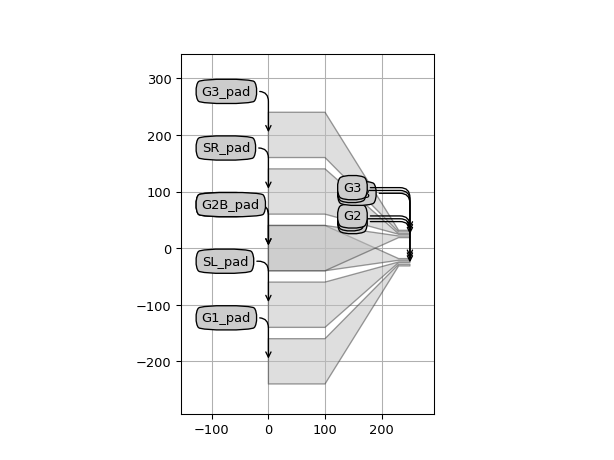SiFab contains the following component to build RF access pads for high-frequency RF probes:

This component allows to generate RF pads with a parametric number of pads. These pads are characterised by a broad section and a narrow section. Their properties are illustrated in the following picture.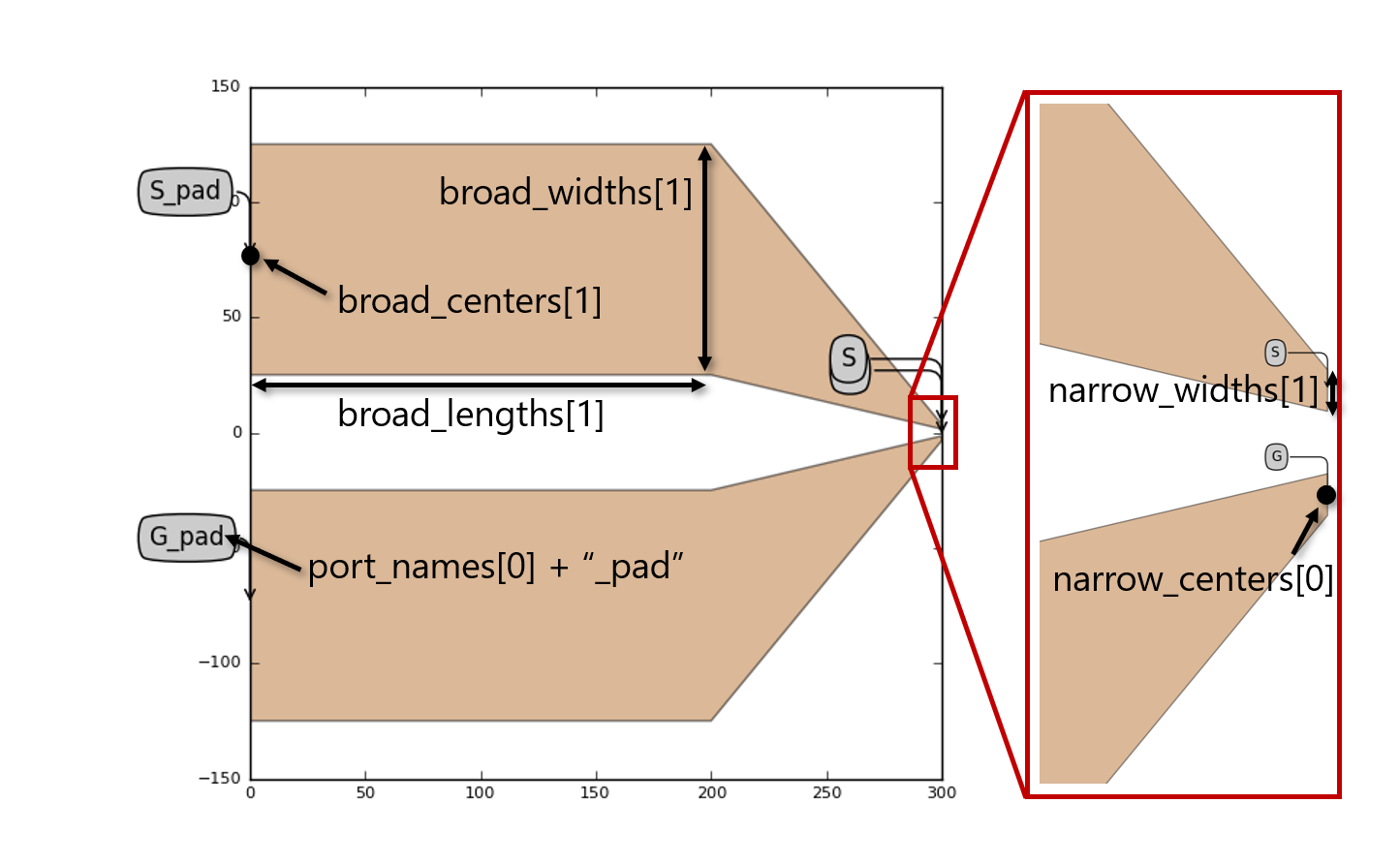Different types of pads can be instantiated easily by adapting the number of layout properties provided, as shown in the examples below.

Reference

Click on the name of the component below to see the complete PCell reference.

 si_fab.all.ProbePad RF access line for high-frequency RF probes.

Example

from si_fab import all as pdk
import numpy as np

# GS
name="GS",
start_widths= * 2,
start_centers=np.array([-0.5, 0.5]) * 150.0,
start_lengths= * 2,
end_widths= * 2,
end_centers=np.array([-0.5, 0.5]) * 5.0,
end_lengths= * 2,
distance=500.0,
port_names=["G", "S"],
)
rf.Layout().visualize(annotate=True)

# GSG
name="GSG",
start_widths= * 3,
start_centers=np.array([-1, 0, 1]) * 150,
start_lengths= * 3,
end_widths=*3,
end_centers=np.array([-1, 0, 1]) * 5,
end_lengths= * 3,
distance=300.0,
port_names=["G1", "S", "G2"],
)
rf.Layout().visualize(annotate=True)

# GSGSG
name="GSGSG",
start_widths= * 5,
start_centers=np.array([-2, -1, 0, 1, 2]) * 150,
start_lengths= * 5,
end_widths=[3, 1, 3, 1, 3],
end_centers=np.array([-2, -1, 0, 1, 2]) * 5,
end_lengths=*5,
distance=300.0,
port_names=["G1", "SL", "G2", "SR", "G3"],
)
rf.Layout().visualize(annotate=True)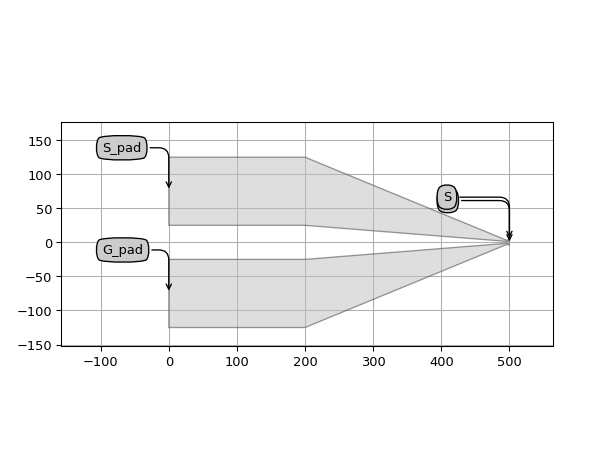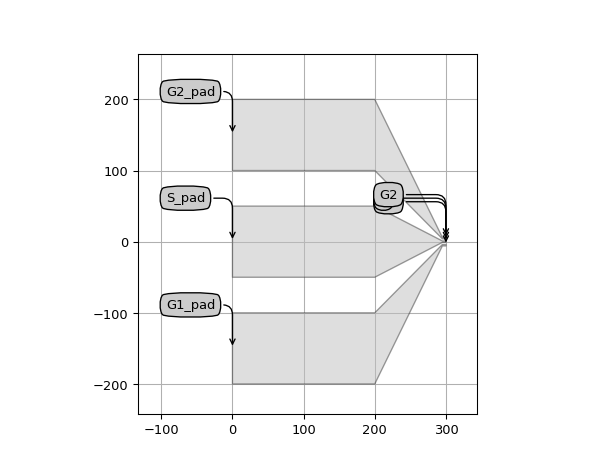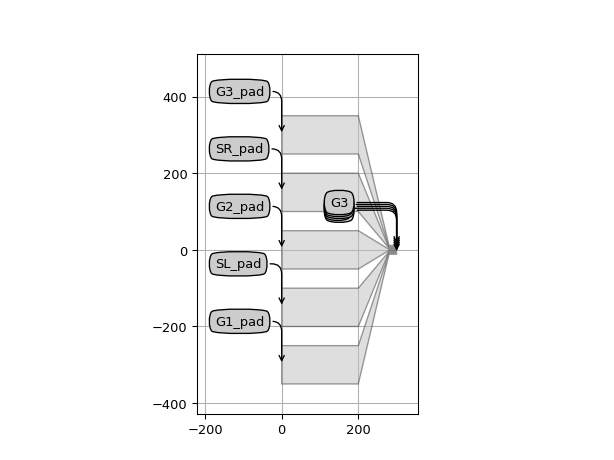This class can also be used to join 2 RF traces into one probe pad, by specifying overlapping start coordinates:

from si_fab import all as pdk
import numpy as np

# GSGGSG